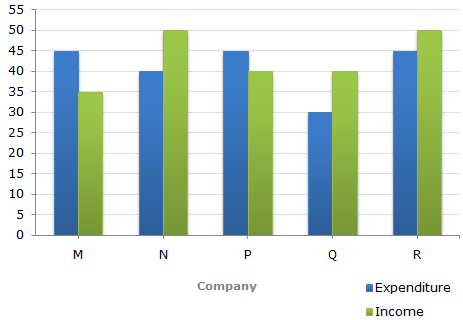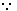# Data Interpretation - Bar Charts

Directions to Solve

The following bar graph shows the Income and Expenditures (in million US \$) of five companies in the year 2001. The percent profit or loss of a company is given by

 % Profit/Loss = Income - Expenditure x 100 Expenditure

Income and Expenditure (in million US \$) of five companies in the year 2001.1.
The companies M and N together had a percentage of profit/loss of?
12% loss
10% loss
10% profit
There was no loss or profit
Explanation:

Total income of Companies M and N together

= (35 + 50) million US \$

= 85 million US \$

Total expenditure of Companies M and N together

= (45 + 40) million US \$

= 85 million US \$.Percent Profit/Loss of companies M and N together

 % Profit/Loss =85 - 85 x 100= 0%. 85

Thus, there was neither loss nor profit for companies M and N together.

2.
In 2001, what was the approximate percentage of profit/loss of all the five Companies taken together?
5% profit
6.5% profit
4% loss
7% loss
Explanation:

Total income of all five companies

= (35 + 50 + 40 + 40 + 50) million US \$

= 215 million US \$.

Total expenditure of all five companies

= (45 + 40 + 45 + 30 + 45) million US \$

= 205 million US \$.% Profit =(215 - 205) x 100% = 4.88%5%. 205

3.
Which company earned the maximum percentage profit in the year 2001?
M
N
P
Q
Explanation:

The percentage profit/loss in the year 2001 for various comapanies are:

 For M =(35 - 45) x 100% = -22.22%   i.e.,   Loss = 22.22%. 45

 For N =(50 - 40) x 100% = 25%   i.e.,   Profit = 25%. 40

 For P =(40 - 45) x 100% = -11.11%   i.e.,   Loss = 11.11%. 45

 For Q =(40 - 30) x 100% = 33.33%   i.e.,   Profit = 33.33%. 30

 For R =(50 - 45) x 100% = 11.11%   i.e.,   Profit = 11.11%. 45

Clearly, the Company Q earned the maximum profit in 2001.

4.
For Company R, if the expenditure had increased by 20% in year 2001 from year 2000 and the company had earned profit of 10% in 2000, what was the Company's income in 2000 (in million US \$)?
35.75
37.25
38.5
41.25
Explanation:

Let the expenditure of Company R in 2000 be x million US \$.

 Then, expenditure of Company R in 2001 =120 x xmillion US \$. 100120x = 45x = 37.5. 100

i.e., expenditure of Company R in 2000 = 37.5 million US \$.

Let the income of Company R in 2000 be I million US \$.

 Then, 10 = (I - 37.5) x 100 [%Profit in 2000 = 10%] 37.5I - 37.5 = 3.75I = 41.25

i.e., Income of Company R in 2000 = 41.25 million US \$.

5.
If the income of Company Q in 2001 was 10% more than its income in 2000 and the Company had earned a profit of 20% in 2000, then its expenditure in 2000 (in million US \$) was?
28.28
30.30
32.32
34.34
Explanation:

Let the income of Company Q in 2001 = x million US \$.

 Then, income of Company in 2001 =110 x xmillion US \$. 100110x = 40x =400. 100 11

 i.e., income of Company Q in 2000 =400million US \$. 11

Let the expenditure of Company Q in 2000 be E million US \$.

 Then, 20 = [(400/11) - E] x 100 [%Profit = 20% ] E20 =400- 1x 100 11EE = 400 x 100 = 30.30. 11 120Expenditure of Company Q in 2000 = 30.30 million US \$.